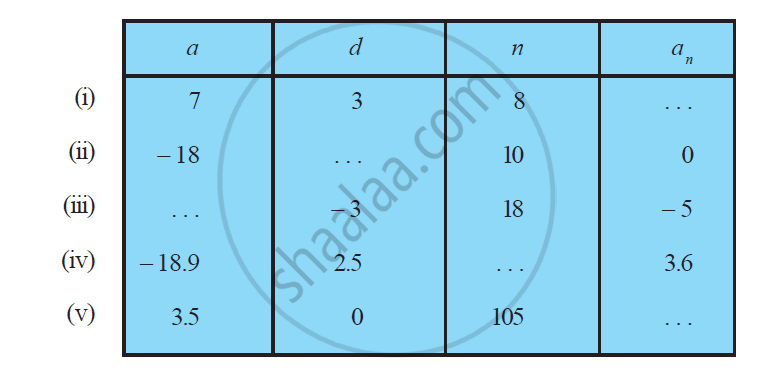# Fill in the blanks in the following table, given that a is the first term, d the common difference and an the nth term of the AP - Mathematics

Fill in the blanks in the following table, given that a is the first term, d the common difference and an the nth term of the AP:#### Solution

(i) a = 7, d = 3, n = 8, an = ?

We know that,

For an A.P. an = a + (n − 1) d

= 7 + (8 − 1) 3

= 7 + (7) 3

= 7 + 21 = 28

Hence, an = 28

(ii) Given that

a = −18, n = 10, an = 0, d = ?

We know that,

an = a + (n − 1) d

0 = − 18 + (10 − 1) d

18 = 9d

d= 18/9 = 2

Hence, common difference, d = 2

(iii) Given that

= −3, n = 18, an = −5

We know that,

an = a + (n − 1) d

−5 = a + (18 − 1) (−3)

−5 = a + (17) (−3)

−5 = − 51

a = 51 − 5 = 46

Hence, a = 46

(iv)

a = −18.9, d = 2.5, an = 3.6, n = ?

We know that,

an = a + (n − 1) d

3.6 = − 18.9 + (n − 1) 2.5

3.6 + 18.9 = (n − 1) 2.5

22.5 = (n − 1) 2.5

(n-1) = (22.5)/2.5

n -1 = 9

n = 10

Hence, n = 10

(v) a = 3.5, d = 0, n = 105, an = ?

We know that,

an = a + (n − 1) d

an = 3.5 + (105 − 1) 0

an = 3.5 + 104 × 0

an = 3.5

Hence, an = 3.5

Concept: nth Term of an AP
Is there an error in this question or solution?

#### APPEARS IN

NCERT Class 10 Maths
Chapter 5 Arithmetic Progressions
Exercise 5.2 | Q 1 | Page 105